# Thread: Dark energy - Accelerating expansion of the universe due to negative mass

1. I apologize for my poor English.
I had a new computer simulation.

We set up each model from the birth of universe to the present, and calculated GPE using computer simulation in each level.

As a result, we could verify that pair creation model of negative mass and positive mass explains inflation of the early universe and decelerating expansion, and present accelerating expansion in time series.

This simulation is showing incredible results.
It not only explains the total energy of the universe, flatness, and the essence (Total zero energy, pair creation of negative energy and positive energy) of the process of birth of the universe, but it explains inflation, decelerating expansion in the early stage, accelerating expansion in the late stage, and dark matter through the only term, negative energy. Moreover, this negative energy is one that is essentially required by the law of energy conservation.

Simulation 1. Dark energy - Accelerating expansion of distant galaxy due to negative mass

Simulation 2. Inflation, decelerating expansion and accelerating expansion with pair creation of negative mass and positive mass

3. Paper: The change of Gravitational Potential Energy and Dark Energy in the Zero Energy Universe.
viXra.org e-Print archive, viXra:1110.0019, The Change of Gravitational Potential Energy and Dark Energy in the Zero Energy Universe

A. Birth of the universe from zero energy state
1) computer simulationFig14. m+=+1 (1,000ea), -m-=-1 (1,000ea),
U++ = -5190.4707907,
U-- = -5308.0373689,
U-+= 10499.2712222,
U_tot = 0.7630625

Total rest mass energy is zero. Total gravitational potential energy () is +0.763., sois almost zero.

We could not make GPE 0 for there were too many particles. Therefore, we simulated dividing the value of(total GPE) into two parts which are when it is little bit bigger than 0 (+0.76306) and when smaller than 0 (-0.53277), and we could gain almost similar results. (Attached 1, 2)

2) Accelerating expansion of the universe (inflation)

It can be confirmed that even though the total energy starts with 0, the universe expands and positive masses combine one another due to attractive interaction among themselves, while negative masses can not form massive mass structure because of repulsive interaction.

The pair creation model of negative mass and positive mass explains energy conservation in times of the birth of the universe and expansion after the birth naturally, and it does not need institution of new mechanism or field like inflaton or inflation itself, and it explains this effect with only gravity.

3) Change of GPE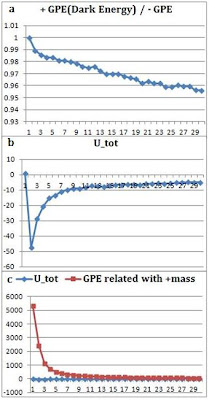Figure15-a.The ratio of negative GPE to positive GPE of the early universe. We can confirm that as the universe expands, (+GPE/-GPE) ratio decreases, andgets to be negative value. Figure15-b. Total GPE of the early universe. Figure15-c.of the early universe and GPE related with positive mass. It looks almost like a straight line for the size ofis relatively small.

The graph above is that the change of GPE related with positive mass anddrawn through graph.

As we have observed activities of only positive masses, GPE related with positive mass () has a significant meaning.

a) Nevertheless the value ofchanges from 0 to negative value, the universe expands for GPE related with positive mass has + value.

b) Note that nevertheless the total energy is 0, GPE related with positive mass has very big positive value, and this value approaches to 0 very rapidly. This explains the dramatic expansion like the early universe inflation and the finish of this inflation mechanism.

c) The thing we can notice by this and the next simulation is that if time goes bit more,and GPE related with positive mass both have negative values, and the universe is converted to the decelerating expansion stage.

d) In order to explain the flatness of the universe, typical researchers assume the inflation mechanism and explain it using this. But Zero Energy Universe does not need institution of new field for it guarantees flatness itself, and additionally, the simulation above means that the accelerating expansion of the early universe can be explained with gravity without instituting new field.

4) Change of GPE related with positive mass andin three initial valueFig16-a. Total rest mass energy=0,(initial value)= +51.79 / +0.76 / -0.53. Time scale=0.5, Step scale(+51.79,-0.53)=200, Step scale(+0.76)=250,
Fig16-b. Change of GPE related with positive mass in three cases

GPE related with positive mass has very big positive value, and this value approaches to 0 very rapidly.

B. GPE among distant galaxies and accelerating expansion
1) When positive mass is spread through relatively large areaFig17.Distant galaxy  The structure that negative mass surrounds galaxy composed of positive mass.= (1 X 500) + (1 X 500) = + 1000, center1(-1000,0,0), center2(+1000,0,0), R=150,= (-1 X 500) +(-1 X 500) = - 1000, negative mass distribution :. We simulated having it has unit mass of positive mass and negative mass.

After the birth of the universe, positive masses bind together by attractive interaction. Meanwhile, negative masses are being almost uniformly distributed because of repulsive interaction. Negative masses are gravitational bounded to massive positive masses (Galaxy or Galaxy cluster) for massive positive mass has attractive effect on negative mass.2.

3.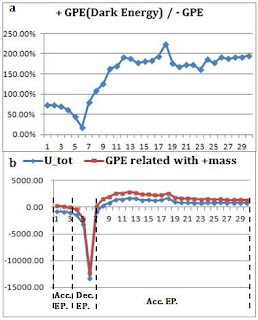Figure18-a.The ratio of +GPE to GPE of distant galaxy. Figure18-b.and GPE related with positive mass on distant galaxy. Note that GPE value related with positive mass changes from positive value to negative value, and to positive value again. This represents acceleration expansiondeceleration expansionacceleration expansion of the universe.

a) The ratio of +GPE to GPE of distant galaxy
i) Early status is that positive GPE is smaller than negative GPE, and thehas negative value. This negative GPE status results from gravitational binding of positive masses.

ii) As time goes by, binding of positive mass increases due to attractive interaction, and the absolute value of negative gravity potential reaches maximum.

iii) The absolute value of negative GPE decreases due to positive mass has a gravitational binding and negative mass does gravitational contraction andis converted to positive value as a result. Center of gravitational contraction of negative masses is galaxy or galaxy cluster.

iv)and GPE related with positive mass both are converted to positive value. Therefore, the universe gets to an era of accelerating expansion again.

v) On the simulation above, we can confirm that +GPE increases 200% the value of GPE, and for we deduce the universal components through GPE, we will guess that repulsive dark energy increases 200% the value of attractive mass energy (matter + dark matter, as a general deduction).

b)and GPE related with positive mass on distant galaxy
i) In the early universe, GPE related with positive mass had very big + value, but this value gets smaller as positive masses binds together and comprise of galaxy structure. On the simulation above, it still has positive value, and so it is in the status of accelerating expansion.

ii) We can notice thatand GPE related with positive mass both are converted to negative value by gravitational binding of positive masses. Therefore, the universe gets to an era of decelerating expansion.

iii) GPE related with positive mass is converted to positive value due to negative mass does gravitational contraction around massive positive mass(Galaxy or Galaxy Cluster). Therefore, the universe gets to an era of accelerating expansion again.

iv) The decelerating expansion and accelerating expansion is naturally explained through pair creation model of negative mass and positive mass, and the conversion from accelerating expansion to decelerating expansion and from decelerating expansion to accelerating expansion is explained in sequence.

v) The conversion from negative value to positive value shall be done more smoothly than the graph above for there exist thousands of billions of galaxies in our universe.Figure19-a.The change of distance and relative speed among distant galaxies. Figure19-b. GPE related with positive mass and negative mass.

c) The change of distance and relative speed among distant galaxies
Massive positive mass is given a birth from the 8th stage due to gravitational contraction. We calculated distance between the two massive positive masses (corresponding to the galaxy or galaxy cluster) and relative speeds of the two from then.

We can notice that there exits positive acceleration, and it corresponds to accelerating expansion.

d) GPE related with positive mass and negative mass
Positive mass and negative mass have different GPE value each other, therefore their movements are different each other.

D. Gravitational contraction due to positive mass and negative mass

1) When positive mass does gravitational contraction
The structure that negative mass surrounds galaxy composed of positive mass.fig24.= (-1 X500)+(-1 X500)= -1000,
negative mass distribution: center1(-1000,0,0), center2(+1000,0,0), within R=220~250.= (+1X500) + (+1X500)= +1000,
positive mass distribution : center1(-1000,0,0), center2(+1000,0,0), within
a)R0-R200, b)R0-R150, c)R0-R100, d)R0-R50

It is shown that as positive mass does gravitational contraction,and GPE value related with positive mass both gradually smaller (negative value).

This means that our universe is converted from accelerating expansion (inflation) in the early universe to decelerating expansion.

2) When negative mass does gravitational contractionfig25.= (500 X1) + (500 X1)= 1000,
positive mass distribution: center1(-1000,0,0), center2(+1000,0,0).= (-1X500) + (-1X500)= -1000,
negative mass distribution : center1(-1000,0,0), center2(+1000,0,0), within
a)R50-R250, b)R50-R200, c)R50-R150, d)R50-R100

It is shown that as negative mass does gravitational contraction around massive positive mass,and GPE value related with positive mass both are bigger.

This means that our universe is converted from decelerating expansion to accelerating expansion (Dark energy effect).

E. Distant six galaxiesfig26. Distant six galaxies.= +100 X 6 = +600.
Each +100 at (±1000,0,0),(0,±1000,0),(0,0,±1000).= (-0.4 X 250) X 6 = - 600
center(±1000,0,0), center(0,±1000,0), center(0,0,±1000) negative mass is spread within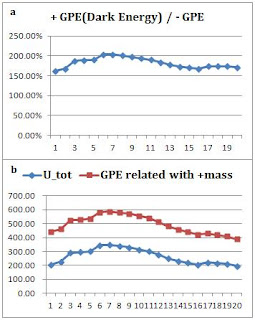fig.27

1) The ratio of +GPE to GPE and GPE related with positive mass on six galaxiesand GPE related with positive mass both have a positive value. Therefore, expansion of universe is accelerating.fig.28

2) The change of distance and relative speed among six galaxies
We can notice that there exits positive acceleration, and it corresponds to accelerating expansion.

F. The change of GPE in the whole time of the universe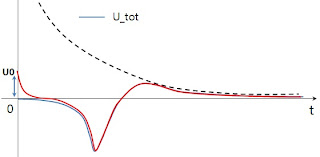fig29. The change ofof universe according to time. Refer to Figure15-b, 16-a, 18-b, 21-b, 21-b, 23-c, 27-b.

2) The change ofof universe according to time.
a) GPE approaches to 0 at last as universe gets larger for it is in proportion to 1/r.

b)can have diverse values in the early universe. But it is assumed that it would have similar graph as time goes.

c) It seems thatmore likely has 0 or positive value. The homogeneous distribution of negative energy and positive energy makeshave positive value (red line).

Although the total GPE starts with 0 or positive value in the early stage, it change to negative value as time goes by, and positive masses forms galaxies binding themselves, and as negative mass does gravitational contraction, it is converted to positive value.

This provides natural explanation about accelerating expansion of the early universe, decelerating expansion in the first half, and accelerating expansion in the second half.fig30.The change ofand GPE value related with positive mass according to time.
The reason of dark energy seems to be constant, is that our universe pass this section (slope) lately 5~7Gyr. Refer to Figure15-b,c,16-a,b,18-b,21-b,23-c,27-b.

3) The change ofand GPE value related with positive mass according to time.

In early universe, Even if the total GPE is 0, the universe can expand in acceleration.
The typical matter we observe in the universe is positive mass, and this is because there are two GPE categories related with positive masses.

Althoughand GPE value related with positive mass can have values that have large difference in the early universe, as time goes by,and GPE value related with positive mass have values and shapes similar to each other.4. III-H. Observation value of WMAP
2)Some interpretation

According to the observance result of WMAP, it is predicted that current dark energy, dark matter, and matter is approximately 72.1%, 23.3%, and 4.6%, respectively.

Now, let's correspond to the GPE as follows.

Matter == Negative GPE
Dark Matter == Negative GPE
Dark Energy == Positive GPE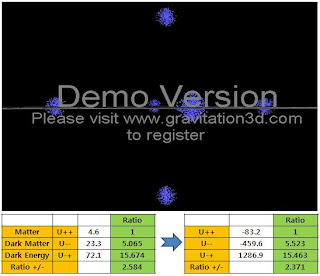fig13. m+ = +100 X 6 = + 600. (±1200,0,0), (0,±1200,0), (0,0,±1200), each 100.= (-0.2 X 500) X 6 = - 600.
Negative mass distribution : center(±1200,0,0), center(0,±1200,0), center(0,0,±1200), negative mass is spread within R=3-120, md=8.

The ratio above is valid between the 3 physical parameters. Case of this ratio being valid was found through simulation.

U++ = -83.2 (1)
U-- = -459.6 (5.523)
U-+ = 1286.9 (15.463)

When we judge the components of the universe, we judge the components by gravitational effect rather than mass energy.

Therefore, when gravitational potential energy U-+ exists larger than gravitational potential energy U which is generated by materials, we will be confused to think that some mass energy bigger than the mass energy of materials exists.

This model starts with a single assumption that is ''There was a pair creation of negative mass and positive mass in the early universe'', and in other word, ''The law of energy conservation came into existence when the universe was birthed.''

This single assumption explains all of the total energy of the universe, flatness, inflation, decelerating expansion, dark energy, and dark mass.

Diverse momentary assumptions that the typical theories (the momentum of inflation, cosmological constant, vacuum energy, dark matter like WIMP) have are not needed, and negative energy is the essential energy to satisfy energy conservation at the time of birth of the universe.

Paper: The change of Gravitational Potential Energy and Dark Energy in the Zero Energy Universe.
viXra.org e-Print archive, viXra:1110.0019, The Change of Gravitational Potential Energy and Dark Energy in the Zero Energy Universe5. Can you or anyone formulate a layman's explanation with a brief description of what negative mass is?6.Originally Posted by icewendigoCan you or anyone formulate a layman's explanation with a brief description of what negative mass is?
No one knows what negative mass is, but there are models that can explain how such a mass would behave :

http://en.wikipedia.org/wiki/Negativ...d.27s_analysis

As you can see this is less than intuitive, but does provide some interesting points for further thought, e.g. the infamous "Diametric Drive". I should note also that, under the theory of relativity, energy and mass are equivalent; it is actually possible to generate regions of space with a negative energy density using the Casimir effect, which is theoretically equivalent to an object with negative mass, albeit a very small one.7.Originally Posted by icewendigoCan you or anyone formulate a layman's explanation with a brief description of what negative mass is?
Hello! icewendigo,

Refer to below simulation.

Negative mass, Dark matter, Dark Energy, Bullet Cluster, Antigravity-1 - YouTube8. how can i understand dark matter/energy?
can i look at it like yeast, using its own source to gain more energy?accelerating expansion, dark energy, dark matter, negative energy, negative mass